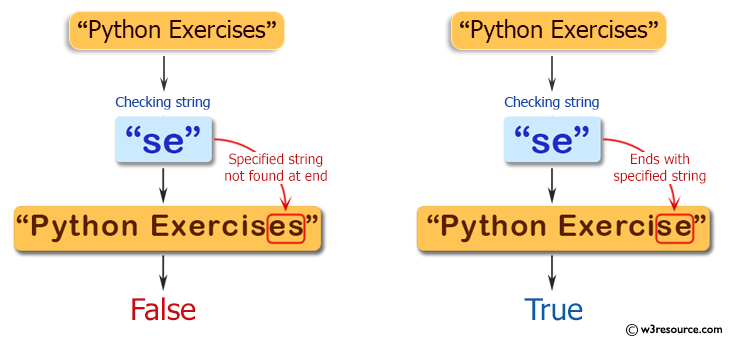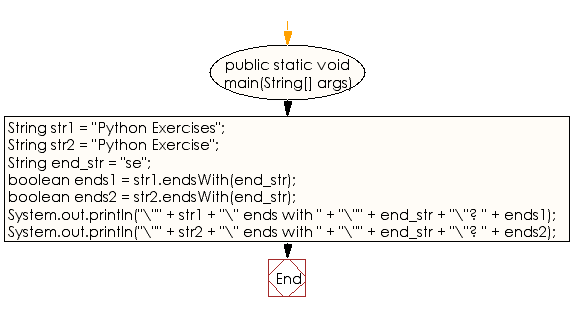﻿ Java exercises: Check whether a given string ends with the contents of another string - w3resource# Java String Exercises: Check whether a given string ends with the contents of another string

## Java String: Exercise-12 with Solution

Write a Java program to check whether a given string ends with the contents of another string.

Pictorial Presentation:Sample Solution:

Java Code:

``````public class Exercise12 {

public static void main(String[] args)
{
String str1 = "Python Exercises";
String str2 = "Python Exercise";

// The String to check the above two Strings to see
// if they end with this value (se).
String end_str = "se";

// Check first two Strings end with end_str
boolean ends1 = str1.endsWith(end_str);
boolean ends2 = str2.endsWith(end_str);

// Display the results of the endsWith calls.
System.out.println("\"" + str1 + "\" ends with " +
"\"" + end_str + "\"? " + ends1);
System.out.println("\"" + str2 + "\" ends with " +
"\"" + end_str + "\"? " + ends2);
}
}
```
```

Sample Output:

```"Python Exercises" ends with "se"? false
"Python Exercise" ends with "se"? true
```

Flowchart:Visualize Java code execution (Python Tutor):

Java Code Editor:

Improve this sample solution and post your code through Disqus

What is the difficulty level of this exercise?

﻿

## Java: Tips of the Day

Directory Content:

Java allows you to get the names of all subdirectories and files in a folder as an array, which can then be sequentially viewed.

```import java.io.*;

public class ListContents {
public static void main(String[] args) {
File file = new File("//home//user//Documents/");
String[] files = file.list();

System.out.println("Listing contents of " + file.getPath());
for(int i=0 ; i < files.length ; i++)
{
System.out.println(files[i]);
}
}
}
```